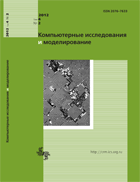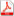Issue 2, 2012 Vol. 4

# All issues

NINE: computer code for numerical solution of the boundary problems for nonlinear differential equations on the basis of CANMpdf (170K)  / List of references

The computer code NINE (Newtonian Iteration for Nonlinear Equation) for numerical solution of the boundary problems for nonlinear differential equations on the basis of continuous analogue of the Newton method (CANM) is presented. Numerov’s finite-difference appproximation is applied to provide the fourth accuracy order with respect to the discretization stepsize. Algorithms of calculating the Newtonian iterative parameter are discussed. A convergence of iteration process in dependence on choice of the iteration parameter has been studied. Results of numerical investigation of the particle-like solutions of the scalar field equation are given.

Keywords: nonlinear differential equations, continuous analogue of the Newton method, finite-difference approximation
Citation in English: Batgerel B., Zemlyanay E.V., Puzynin I.V. NINE: computer code for numerical solution of the boundary problems for nonlinear differential equations on the basis of CANM // Computer Research and Modeling, 2012, vol. 4, no. 2, pp. 315-324
Citation in English: Batgerel B., Zemlyanay E.V., Puzynin I.V. NINE: computer code for numerical solution of the boundary problems for nonlinear differential equations on the basis of CANM // Computer Research and Modeling, 2012, vol. 4, no. 2, pp. 315-324
DOI: 10.20537/2076-7633-2012-4-2-315-324
Views (last year): 1. Citations: 1 (RSCI).

Full-text version of the journal is also available on the web site of the scientific electronic library eLIBRARY.RU

The journal is included in the Russian Science Citation Index

The journal is included in the List of Russian peer-reviewed journals publishing the main research results of PhD and doctoral dissertations.

International Interdisciplinary Conference "Mathematics. Computing. Education"

The journal is included in the RSCI

Indexed in Scopus